# 在UG中绘制变螺距螺旋线的方法

 图1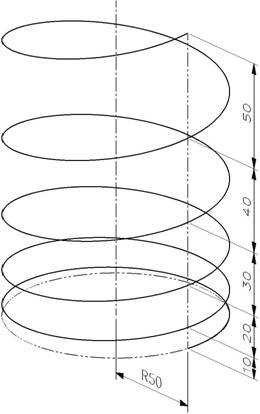1、用“表达式”

Start_pitch=10                                 //起始圈螺距

End_pitch=50                                //终止圈螺距

Turns=5                                        //螺旋线圈数

mean_pitch=(Start_pitch+End_pitch)/2        //平均螺距

height=Turns*mean_pitch                        //螺旋线高度

t=0                                            //系统变量

x=t*height

x1=0

x2=mean_pitch

x3=height-mean_pitch

x4=height

z1=0

z2=Start_pitch

z3=height-End_pitch

z4=height

zt1=(((x-x2)*(x-x3)*(x-x4)/((x1-x2)*(x1-x3)*(x1-x4))))*z1

zt2=(((x-x1)*(x-x3)*(x-x4)/((x2-x1)*(x2-x3)*(x2-x4))))*z2

zt3=(((x-x1)*(x-x2)*(x-x4)/((x3-x1)*(x3-x2)*(x3-x4))))*z3

zt4=(((x-x1)*(x-x2)*(x-x3)/((x4-x1)*(x4-x2)*(x4-x3))))*z4

zt=zt1+zt2+zt3+zt4                             //Z规律

2、用“缠绕/展开曲线”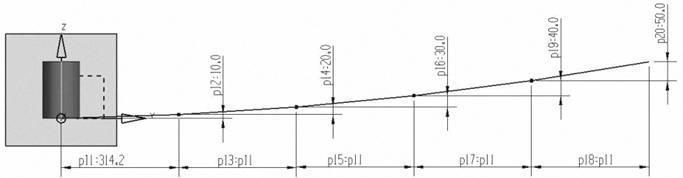首先做一个φ100×150的圆柱体（定位点在原点，轴向为Z轴，作为被缠绕体，控制了变螺距螺旋线的直径、高度），然后创建一个与圆柱体相切且与X轴垂直的基准平面，用“草图”在此基准面上绘制变螺距螺旋线的展开曲线（水平尺寸为每圈螺旋线的圆周长，垂直尺寸为每圈螺旋线的高度）。如图2所示。

 图2

3、用“扫掠”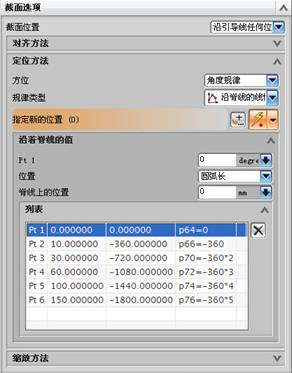在“扫掠”对话框中的“截面选项”中设置，方位为“角度规律”，规律类型为“沿脊线的线性”。在引导线上依次选择6个特殊位置（在扫掠过程中，截面线从起始到每圈螺旋线结束，螺旋线的高度），并定义截面线从起始位置扫掠至此的角度。其相关设置如图3所示。

 图3

 图4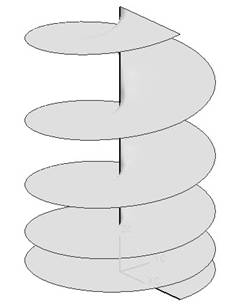4、用“规律延伸”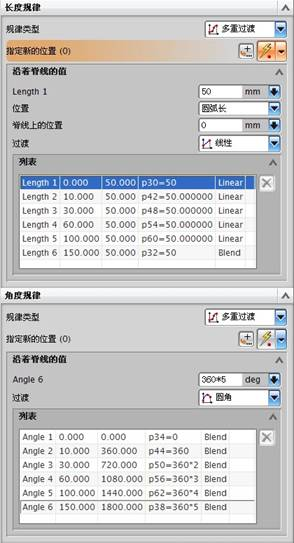以原点为起点作一条平行于Z轴的直线（长度150mm）。

 图5